### Excel Percentages and Differences# Percentages and Differences

Some of the most common calculations on Excel worksheets are Percentages and Differences. Percentages: Show one number as a percentage of another, or a percentage of the Grand total. We have to do these formulas constantly. Differences are just as common. Budget vs Actual, Plan vs Achieved, Current Year vs Previous Year etc. These formulas are the meat and drink of Excel worksheets.

Usually the formulas you need are quite straightforward: divisions for percentages and a minus sign to take one value from another. But there are pitfalls for the unwary which we shall explore. Read on…

## Comparison

This is all about showing one number as a percentage of another number. In the example, we need to show Costs as a percentage of Revenue. In your formula, divide the smaller number by the larger number and then copy it down the column.

In the example, the formula’s really easy, =B2/C2

The formula is correct but the results are shown as decimals, Excel cells typically have a General number format. We need to show those results in column D as percentages so that they are easier for our audience to understand. To format the percentage cells either use the Percent Style control on the Home tab or right-click and choose Format Cells, then apply the Percentage category in the Number tab.

Most people find percentages much easier to comprehend than decimals.

Percentage formats multiply the cell value by 100 and displays the result with a percent symbol.

## As a Percentage of the Total

This is a very popular calculation, showing a number as a percentage of the whole. Again, it works on the principle that people can interpret percentages far easier than they can raw numbers.

We’ve divided the Revenue for the first item by the grand total and it’s worked fine for the first calculation. But the formulas go haywire when copied down.

It’s obvious what the problem is when we show our formulas. The divisor, B10—the Grand Total, needs to be constant in our formulas. Time for dollar signs!

Normal cell references like B10 are relative. This means they change when they are copied down or across. To fix a cell reference in a formula, you need an absolute reference, like this \$B\$10.

You don’t have to show the formulas in the cells but it helps you see what you’re doing. Click the Show Formulas control on the Formulas tab.

To enter the dollar signs, either type them in or click the B10 reference in your formula and then press the F4 key. (Try Command+T if you’re on a Mac)

When you’ve fixed the cell reference in the first formula you can copy it down. Click Show Formulas again and then apply the percentage number format to your results.

## Percentage Change, the Power of One

Usually, it’s considered bad practice to include constants (fixed numbers) in your formulas but there are a few exceptions when we’re using that magic number, Number One! In this first calculation we need to show the percentage change between two numbers, namely the percentage increase or decrease between Actual and Budget.

Here’s the simple and obvious method. Calculate the difference between Actual and Budget (B2C2 in the formula) and then divide the result by the Budget (C2 in the formula) Of course, we need to have brackets around the minus calculation as otherwise the division would be calculated first.

The formula works but it is too complicated as we have two references to C2 and that can’t be right.

And, indeed, it isn’t necessary to refer to the same cell twice but we’ll need a bit of magic mathematics to simplify the formula and force out the duplicated reference.

The original formula was =(B2C2)/C2, which we can reduce to: =B2/C2-1

Can you see what I did there? If you can’t follow it, have a look at the algebra of the formula in the following section.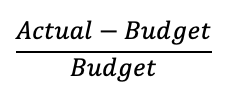Here’s the algebra of the original formula. Actual minus Budget to calculate the difference. And then all divided by the Budget to calculate the percentage.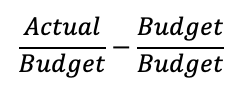Firstly expand the formula: Actual divided by Budget minus Budget divided by Budget. This gives exactly the same result as the original.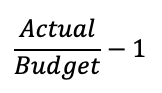And finally, reduce the formula. A number divided by itself is always one. So, we replace Budget divided by Budget with one. Much simpler and far more efficient.

## Percentage Increase, the Power of One

Here’s another calculation where it’s all too easy to create a formula which is easy to understand but too complicated. In the example we have Prices in column B, a Percentage increase or decrease in column C and we need to calculate the new price in column D.

Here’s my first attempt at the formula, it has the original price (B2 in the formula) plus the amount of the increase or decrease (B2*C2 in the formula):

=B2+B2*C2

Easy to understand but inefficient as there are two references to B2.

Once again, the formula needs to be simplified, it now becomes the original price (B2 in the formula) multiplied by one plus the amount of the increase or decrease:

=B2*(1+C2)

See the reduction algebra below.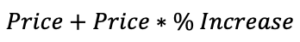The first attempt was the original price plus the amount of the percentage increase to calculate the new price.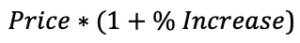To restate a value you just multiply it by one. Only, in this case, you increase the multiplier by the amount of the percentage increase. Dead easy, but just not obvious.

That’s all for now. I hope I managed to give you a few ideas on calculating Excel percentages and differences. Best of luck with all your Excel formulas!To get the best out of Excel formulas you’ll need to have a good, working knowledge of Excel functions. Come along on our dedicated Excel functions course and discover what Excel formulas can do for you.

Call 020 7920 9500 now for details.